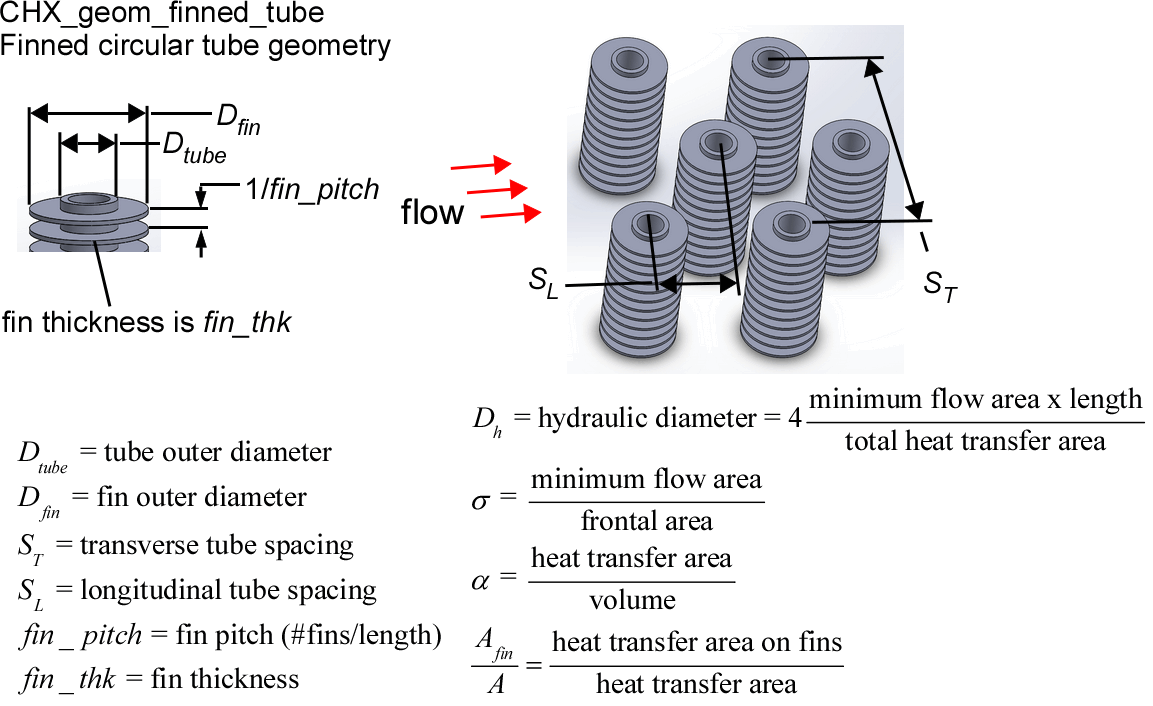Contents - Index

Finned circular tubes geometryThe procedure:

CHX_geom_finned_circular_tube(TypeHX\$: D_tube, D_fin, S_T, S_L, fin_pitch, fin_thk, D_h, sigma, alpha, A_fin\A)

Provides the geometric data associated with a finned circular tube compact heat exchanger surface.  These data are from Kays and London (1994).

Inputs:

The only input is the string identifying the geometry TypeHX\$

CF-7.34 : 'fc_tubes_sCF-734'

CF-8.72 : 'fc_tubes_sCF-872'

CF-8.72(c) : 'fc_tubes_sCF-872c'

CF-7.0-5/8J : 'fc_tubes_sCF-70-58J'

CF-8.7-5/8J(a) : 'fc_tubes_sCF-87-58Ja'

CF-8.7-5/8J(b) : 'fc_tubes_sCF-87-58Jb'

CF-9.05-3/4J(a) : 'fc_tubes_sCF-905-34Ja'

CF-9.05-3/4J(b) : 'fc_tubes_sCF-905-34Jb'

CF-9.05-3/4J(c) : 'fc_tubes_sCF-905-34Jc'

CF-9.05-3/4J(d) : 'fc_tubes_sCF-905-34Jd'

CF-9.05-3/4J(e) : 'fc_tubes_sCF-905-34Je'

CF-8.8-1.0J(a) : 'fc_tubes_sCF-88-10Ja'

CF-8.8-1.0J(b) : 'fc_tubes_sCF-88-10Jb'

8.0-3/8T : 'fc_tubes_80_38T'

7.75-5/8T : 'fc_tubes_775-58T'

Outputs:

D_tube = tube outer diameter (m or ft)

D_fin = fin outer diameter (m or ft) (this output is set to -1 for non-circular fins)

S_T = transverse tube spacing (m or ft)

S_L = longitudinal tube spacing (m or ft)

fin_pitch = # fins per length (fins/m or fins/ft)

fin_thk = fin thickness (m or ft)

D_h = hydraulic diameter defined as 4 x minimum flow area x length/heat transfer area (m or ft)

sigma = minimum flow area/frontal area

alpha = heat transfer area/volume (m^2/m^3 or ft^2/ft^3)

A_fin\A = heat transfer area on fins/heat transfer area

Example

\$UnitSystem SI Mass J K Pa

TypeHX\$='fc_tubes_sCF-88-10Ja'

Call chx_geom_finned_circular_tube(TypeHX\$: D_tube, D_fin, S_T, S_L, fin_pitch, fin_thk, D_h, sigma, alpha, A_fin\A)

{Solution is:

D_tube = 0.026 [m]

D_fin = 0.0441 [m]

S_T = 0.0498 [m]

S_L = 0.0524 [m]

fin_pitch = 346.5 [1/m]

fin_thk = 0.00031 [m]

D_h = 0.00598 [m]

sigma = 0.439

alpha = 299 [m^2/m^3]

A_fin\A = 0.825}

Related procedures include:

Nondimensional Functions

Coefficient of Heat Transfer

Pressure Drop

Compact Heat Exchangers